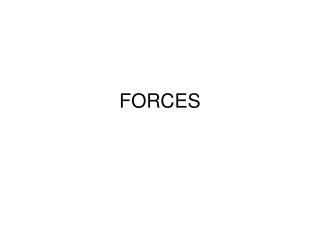DownloadDownload PresentationFORCES

FORCES

Download PresentationFORCES

- - - - - - - - - - - - - - - - - - - - - - - - - - - E N D - - - - - - - - - - - - - - - - - - - - - - - - - - -
Presentation Transcript

1. FORCES

2. Which vehicle exerts a greater force ― the tow truck or the car? ISP 209 - 2B

3. What is a force? • A push or a pull • When one object pushes or pulls another object, you say that the first object exerts a force on the second object. • Let’s practice. What forces are at play around you?

4. What forces are exerted?

5. What forces are exerted? Playing catch with a medicine ball

6. Playing catch with a medicine ball A B A throws the ball and B catches it. four forces When A throws the ball he exerts a force on the ball (toward the right) and the ball exerts a force on him so he recoils (toward the left). When B catches the ball he exerts a force on the ball (toward the left to stop it) and the ball exerts a force on him so he is knocked back (toward the right). ISP 209 - 2B

7. Let’s take a minute to discuss…. Scalars and Vectors • Are both quantities • BUT….. • Scalars are fully described by magnitude (magnitude= number) • Vectors are fully described by both magnitude(number) and direction

8. What is force categorized as? • Force is described by the strength and direction in which it acts. • Therefore, • Force is a VECTOR

9. The strength of force is measured in… • The SI unit called the Newton (N) • One Newton is the amount of force required to give a 1-kg mass an acceleration of 1 m/s/s. • Thus, the following unit equivalency can be stated:

10. Combining Forces • Often, more than one force acts on an object at a time. • Net force is the combination of all forces acting on the object • What does the net force determine? • Motion • direction

11. Net force Practice • For each diagram, write the net force and direction. HINT: The net force always acts in the direction of the greater force.

12. Balanced forces • Equal forces acting on one object in opposite directions.

13. Balanced Forces • Balanced forces result in equilibrium • How can I make this box in equilibrium?

14. Unbalanced Forces • Unbalanced forces result when the forces exerted on an object are not equal. • Unbalanced forces acting on an object result in a net force and cause a change in the object’s motion.

15. Free-Body Diagrams • Free-body diagrams are diagrams used to show the relative magnitude and direction of all forces acting upon an object in a given situation.

16. Draw a free body diagram • A physics book is sitting on a table and is pushed 10 N to the right. The opposing frictional force is 7 N.

17. The horse pulls the cart with a force A (to the right). According to Newton, the cart pulls the horse with a force –A (to the left). A puzzle Horse and Cart So how can they start moving, or accelerate? Resolution: Consider each part separately, and don’t forget that there are other forces acting. ISP 209 - 2B

18. Homework • Read Introduction of Forces notes • Give 3 examples of force for each of the following: (YOUR OWN EXAMPLES!) • Gravity • Tension • Friction • Drag/Air resistance